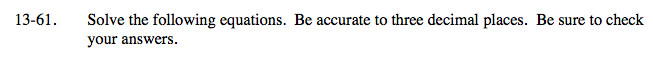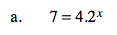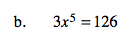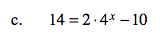### Home > A2C > Chapter 13 > Lesson 13.1.3 > Problem13-61

13-61.
1. Solve the following equations. Be accurate to three decimal places. Be sure to check your answers. Homework Help ✎

1. 7 = 4.2x

2. 3x5 = 126

3. 14 = 2 · 4x − 10ln 7 = x ln 4.2

1.356$x=\sqrt{42}$See part (a).